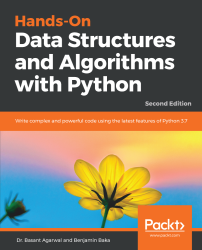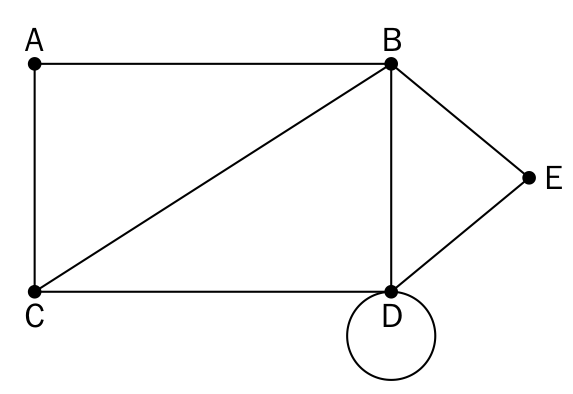•#### Hands-On Data Structures and Algorithms with Python - Second Edition#### Overview of this book

Data structures allow you to store and organize data efficiently. They are critical to any problem, provide a complete solution, and act like reusable code. Hands-On Data Structures and Algorithms with Python teaches you the essential Python data structures and the most common algorithms for building easy and maintainable applications. This book helps you to understand the power of linked lists, double linked lists, and circular linked lists. You will learn to create complex data structures, such as graphs, stacks, and queues. As you make your way through the chapters, you will explore the application of binary searches and binary search trees, along with learning common techniques and structures used in tasks such as preprocessing, modeling, and transforming data. In the concluding chapters, you will get to grips with organizing your code in a manageable, consistent, and extendable way. You will also study how to bubble sort, selection sort, insertion sort, and merge sort algorithms in detail. By the end of the book, you will have learned how to build components that are easy to understand, debug, and use in different applications. You will get insights into Python implementation of all the important and relevant algorithms.
PrefaceFree Chapter
Python Objects, Types, and ExpressionsPython Data Types and StructuresPrinciples of Algorithm DesignLists and Pointer StructuresStacks and QueuesTreesHashing and Symbol TablesGraphs and Other AlgorithmsSearchingSortingSelection AlgorithmsString Algorithms and TechniquesDesign Techniques and StrategiesImplementations, Applications, and ToolsOther Books You May Enjoy# Graphs

A graph is a set of vertices and edges that form connections between the vertices. In a more formal approach, a graph G is an ordered pair of a set V of vertices and a set E of edges, given as G = (V, E) in formal mathematical notation.

An example of a graph is given here:Let's discuss some of the important definitions of a graph:

• Node or vertex: A point or node in a graph is called a vertex, which is usually represented in a graph by a dot. In the preceding diagram, the vertices or nodes are A, B, C, D, and E.
• Edge: This is a connection between two vertices. The line connecting A and B is an example of an edge in the preceding graph.
• Loop: When an edge from a node is incident on itself, that edge forms a loop.
• Degree of a vertex: The total number of edges that are incident on a given vertex is called the degree of that vertex. For example, the degree of the B vertex...